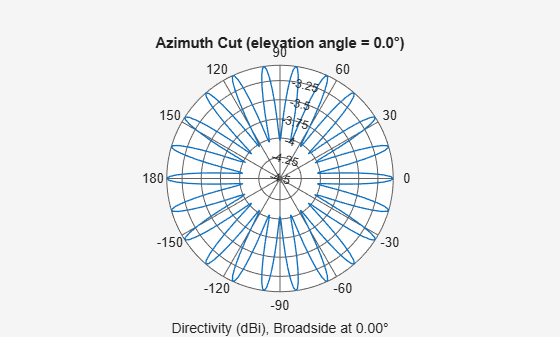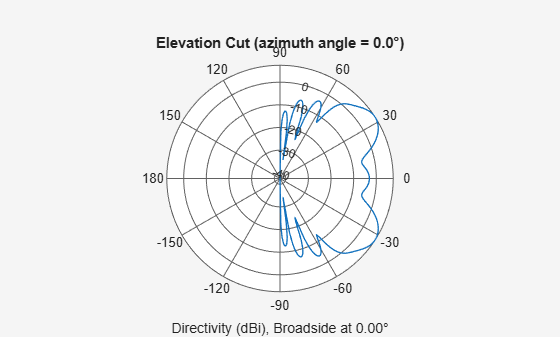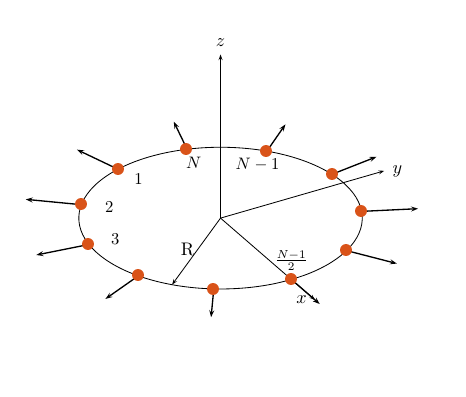# phased.UCA

Uniform circular array

## Description

The `phased.UCA` System object™ creates a uniform circular array (UCA). A UCA is formed from identical sensor elements equally spaced around a circle.

To compute the response for the array for specified directions:

1. Define and set up your uniform circular array. See Construction.

2. Call `step` to compute the response according to the properties of `phased.UCA`. The behavior of `step` is specific to each object in the toolbox.

### Note

Starting in R2016b, instead of using the `step` method to perform the operation defined by the System object, you can call the object with arguments, as if it were a function. For example, `y = step(obj,x)` and `y = obj(x)` perform equivalent operations.

## Construction

`sUCA = phased.UCA` creates a uniform circular array (UCA) System object, `sUCA`, consisting of five identical isotropic antenna elements,`phased.IsotropicAntennaElement`. The elements are equally spaced around a circle of radius 0.5 meters.

`sUCA = phased.UCA(Name,Value)` creates a System object, `sUCA`, with each specified property Name set to the specified Value. You can specify additional name-value pair arguments in any order as (`Name1,Value1`,...,`NameN,ValueN`).

`sUCA = phased.UCA(N,R)` creates a UCA System object, `sUCA`, with the `NumElements` property set to `N` and the `Radius` property set to `R`. This syntax creates a UCA consisting of isotropic antenna elements, `phased.IsotropicAntennaElement`.

`sUCA = phased.UCA(N,R,Name,Value)` creates a UCA System object, `sUCA`, with the `NumElements` property set to `N`, the `Radius` property set to `R`, and other specified property Names set to the specified Values.

## Properties

expand all

Sensor array element, specified as a Phased Array System Toolbox antenna or microphone element System object. You can specify antenna elements which do or do not support polarization.

Example: `phased.ShortDipoleAntennaElement()`

Number of array elements, specified as an integer greater than one.

Example: `3`

Array radius, specified as a positive scalar in meters.

Example: `2.5`

Array normal direction, specified as one of `'x'`, `'y'`, or `'z'`. UCA elements lie in a plane orthogonal to the array normal direction. Element boresight vectors lie in the same plane and point radially outward from the origin.

ArrayNormal Property ValueElement Positions and Boresight Directions
`'x'`Array elements lie on the yz-plane. All element boresight vectors lie in the yz-plane and point outward from the array center.
`'y'`Array elements lie on the zx-plane. All element boresight vectors lie in the zx-plane and point outward from the array center.
`'z'`Array elements lie on the xy-plane. All element boresight vectors lie in the xy-plane and point outward from the array center.

Example: `'y'`

Element tapering or weighting, specified as a complex-valued scalar, 1-by-N row vector, or N-by-1 column vector. The quantity N represents the number of elements of the array. Tapers, also known as weights, are applied to each sensor element in the sensor array and modify both the amplitude and phase of the received data. If `'Taper'` is a scalar, the same taper value is applied to all element. If `'Taper'` is a vector, each taper value is applied to the corresponding sensor element.

Example: `[1 2 3 2 1]`

## Methods

 collectPlaneWave Simulate received plane waves directivity Directivity of uniform circular array getElementNormal Normal vectors for array elements getElementPosition Positions of array elements getElementSpacing Spacing between array elements getNumElements Number of elements in array getTaper Array element tapers isPolarizationCapable Polarization capability pattern Plot UCA array pattern patternAzimuth Plot UCA array directivity or pattern versus azimuth patternElevation Plot UCA array directivity or pattern versus elevation step Output responses of array elements viewArray View array geometry
Common to All System Objects
`release`

Allow System object property value changes

## Examples

collapse all

Create an 11-element uniform circular array (UCA) having a 1.5 m radius and operating at 500 MHz. The array consist of short-dipole antenna elements. First, display the vertical component of the response at 45 degrees azimuth and 0 degrees elevation. Then plot the azimuth and elevation directivities.

```antenna = phased.ShortDipoleAntennaElement(... 'FrequencyRange',[50e6,1000e6],... 'AxisDirection','Z'); array = phased.UCA('NumElements',11,'Radius',1.5,'Element',antenna); fc = 500e6; ang = [45;0]; resp = array(fc,ang); disp(resp.V)```
``` -1.2247 -1.2247 -1.2247 -1.2247 -1.2247 -1.2247 -1.2247 -1.2247 -1.2247 -1.2247 -1.2247 ```

Display the azimuth directivity pattern at 500 MHz for azimuth angles between -180 and 180 degrees.

```c = physconst('LightSpeed'); pattern(array,fc,[-180:180],0,'Type','directivity','PropagationSpeed',c)```Display the elevation directivity pattern at 500 MHz for elevation angles between -90 and 90 degrees.

`pattern(array,fc,,[-90:90],'Type','directivity','PropagationSpeed',c)`## Algorithms

A UCA is formed from N identical sensor elements equally spaced around a circle of radius R. The circle lies in the xy-plane of the local coordinate system whose origin lies at the center of the circle. The positions of the elements are defined with respect to the local array coordinate system. The circular array lies in the xy-plane of the coordinate system. The normal to the UCA plane lies along the positive z-axis. The elements are oriented so that their main response directions (normals) point radially outward in the xy-plane.If the number of elements of the array is odd, the middle element lies on the x-axis. If the number of elements is even, the midpoint between the two middle elements lies on the x-axis. For an array of N elements, the azimuth angle of the position of the nth element is given by

The azimuth angle is defined as the angle, in the xy-plane, from the x-axis toward the y-axis. The elevation angle is defined as the angle from the xy-plane toward the z-axis. The angular distance between any two adjacent elements is 360/N degrees. Azimuth angle values are in degrees. Elevation angles for all array elements are zero.

## References

 Brookner, E., ed. Radar Technology. Lexington, MA: LexBook, 1996.

 Van Trees, H. Optimum Array Processing. New York: Wiley-Interscience, 2002, pp. 274–304.